# Assignment #53: Randomness

## Code

```/// Name: Kiran O'Farrell
/// Program Name: Randomness
/// File Name: Randomness.java
/// Date Completed: 11/30/15

import java.util.Random;

public class Randomness
{
public static void main ( String[] args )
{
Random r = new Random(11111);

int x = 1 + r.nextInt(10);

System.out.println( "My random number is " + x );

System.out.println( "Here are some numbers from 1 to 5!" );
System.out.print( 1 + r.nextInt(5) + " " );
System.out.print( 1 + r.nextInt(5) + " " );
System.out.print( 1 + r.nextInt(5) + " " );
System.out.print( 1 + r.nextInt(5) + " " );
System.out.print( 1 + r.nextInt(5) + " " );
System.out.print( 1 + r.nextInt(5) + " " );
System.out.println();

System.out.println( "Here are some numbers from 1 to 100!" );
System.out.print( 1 + r.nextInt(100) + "\t" );
System.out.print( 1 + r.nextInt(100) + "\t" );
System.out.print( 1 + r.nextInt(100) + "\t" );
System.out.print( 1 + r.nextInt(100) + "\t" );
System.out.print( 1 + r.nextInt(100) + "\t" );
System.out.print( 1 + r.nextInt(100) + "\t" );
System.out.println();

int num1 = 1 + r.nextInt(10);
int num2 = 1 + r.nextInt(10);

if ( num1 == num2 )
{
System.out.println( "The random numbers were the same! Weird." );
}
if ( num1 != num2 )
{
System.out.println( "The random numbers were different! Not too surprising, actually." );
}
}
}

// With the "1 +" at the beginning of the line removed, the random number generator yields a range of 0-4, not 1-5.
// When the "1 +" is changed to "3 +", it generates numbers in a range of 3-7.
// When the random number seed was set to a specific value, the "random" numbers were the same each time.
```

### Pictures of the output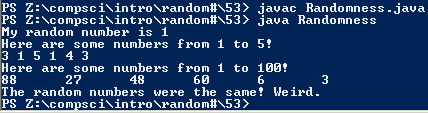The program without code alterations.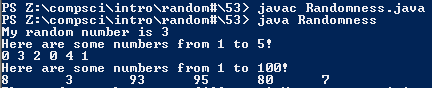The program with the "1 +" removed.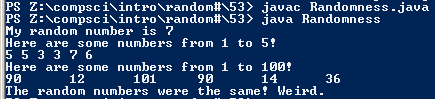The program with the "1 +" changed to "3 +"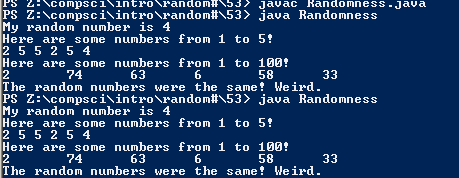The program with the specific seed given in the assignment.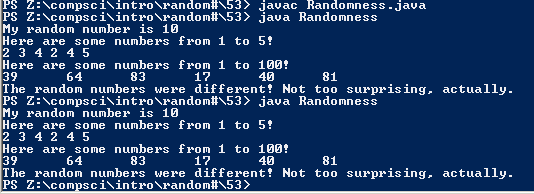The program with a seed I chose.